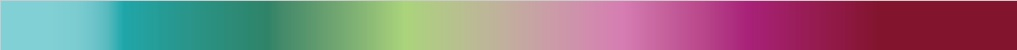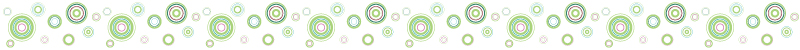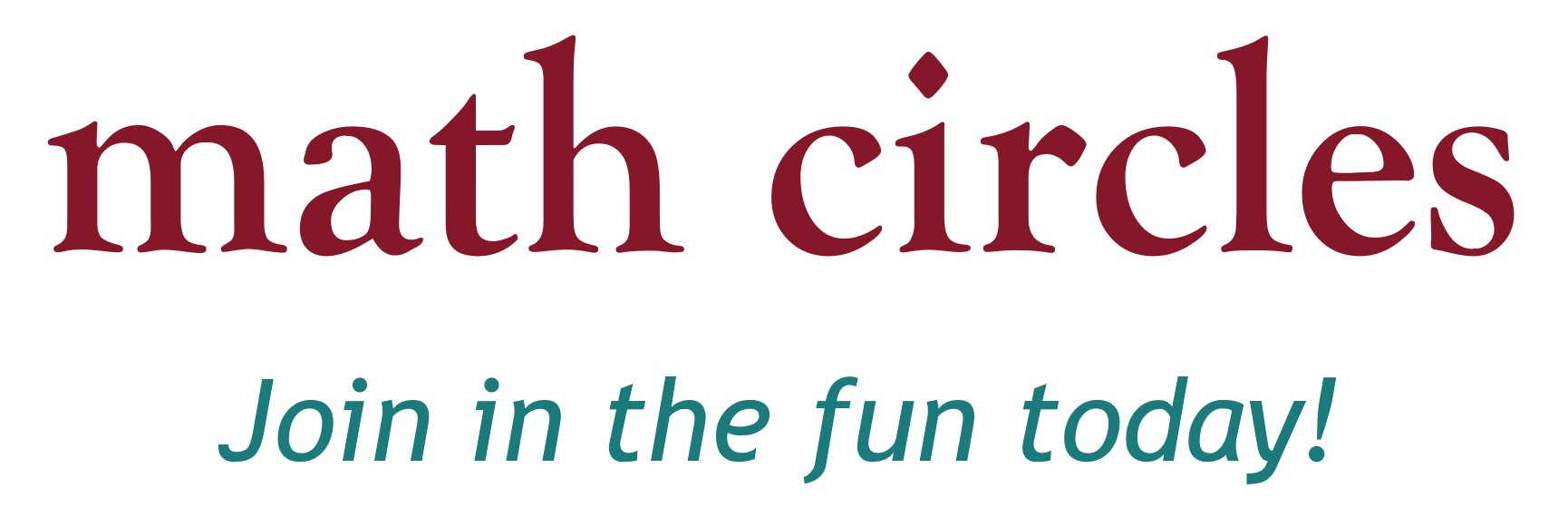Home About Us School Visits Elementary Schools Junior High Schools Senior High Schools Events at Dalhousie Contact Us
Senior High Schools

The below is a list of presentations appropriate for senior high school classes, in alphabetical order. If you would like us to come and give any of these presentations in your classroom for free, please use the contact form on the School Visits page.

Bothersome BrainteasersHas it been a real long time since you have 'troubled' yur brain in finding the right answer to a question? In this talk, you will experience four different brainteasers on shapes and patterns to tease your brain and have a good laugh too!

Outcomes:

• TBA.

CryptographyWhy are prime numbers so important? How is our credit card information kept secret when we make purchases online? By introducing modular arithmetic we will answer these questions and look at the evolution of cryptography.

Outcomes:

• Understand the basic setting of cryptography.
• Understand why digital security is so important.
• Understand the different aspects of digital security.
• Use life knowledge to solve math problems.

Eulerian Circuits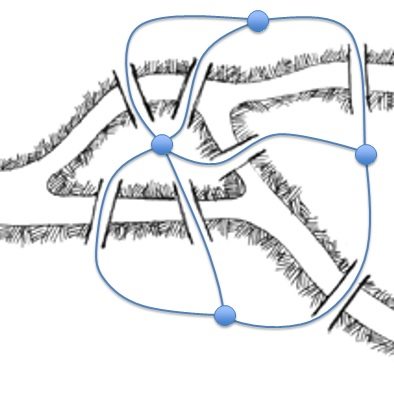In this talk, students will be asked to find a route through the historic city of Königsberg so that they cross every bridge exactly once. This problem spawned an area of mathematics called graph theory, which makes this problem (and many others) much easier to solve! This talk serves as a gentle introduction to graph theory and its applications.

Outcomes:

• Solve problems using graphs.
• Learn definitions involving graphs.
• Look into different types of graphs.
• Try to deduce mathematical theorems.
• Understand why graph theory is so important in real life.

Fibonacci & the Golden RatioIn this talk students discover the relationship between the Golden Ratio and Fibonacci numbers. We also learn why the Golden Ratio is found throughout nature and listen to Fibonacci number inspired music.

Outcomes:

• What can happen when you sum numbers with a certain pattern.
• Look into a spatial math sequence and a special number, that appears in nature a lot and is now used in everyday life.
• Math and art.
• Math and music.
• Count the number of solutions to a problem.

Fractals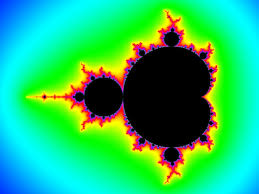Through artwork and the self similarity of nature, we will investigate the mathematical structure of fractals. Fractals are presented through active play of the Chaos game. The class is asked to work as a team to make decisions that drive the movements of volunteers at the front of the room. The class then records this activity on their own sheets and continues the game, resulting in a fractal pattern, on their own sheets.

Outcomes:

• What is Pascal's Triangle and an application of it.
• How to construct fractals.
• Where we find fractals in nature.
• Manipulate linear and quadratic polynomials, to understand complex number computations.

Graph ColouringThis talk introduces students to graph theory. We look at the four colour problem, how graph colouring can be used to solve scheduling problems and even lead students through an activity that will classify what graphs can be coloured with 2 colours.

Outcomes:

• Solve problems using graphs.
• Look into different types of graphs.
• Try to deduce mathematical theorems.
• Understand why graph theory is so important in real life.
• Look into the known problem of map colouring.
• Applications to scheduling problems.

InfinityWhile the topic of Infinity can be an intimidating one for many, the attempt was to approach it through real-life situations that are extrapolated into the infinite. We start by telling students that we have a hotel with an infinite number of rooms, and every room is full. We thenask, can we fit 1 more person? 10 more people? An infinite number of people? In this talk we explore the infinite and by answering these questions, introduce students to transfinite arithmetic

Outcomes:

• Get a better understanding of the notion of infinity.
• Look at the famous problem of Hilbert's motel.

Logic and Reasoning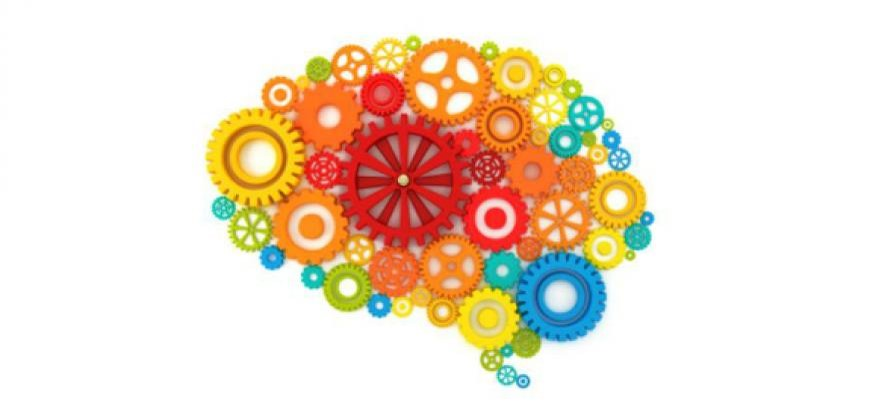In this talk we will introduce students to formal logic, apply it to some fun activities like the "wolf, goat, cabbage" problem, introduce the idea of 'proof' in a nonthreatening way and learn that math is not all numbers!

Outcomes:

• Prove a conjecture, using deductive reasoning.
• Provide and explain a counterexample to disprove a given conjecture.
• Solve a contextual problem involving inductive or deductive reasoning.
• Explain and verify a strategy to solve a puzzle or to win a game.
• Prove algebraic and number relationships.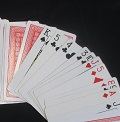In this talk, we will introduce the students to different cards tricks and explain the math behind them.

Outcomes:

• TBA.

Million Dollar Hat Problem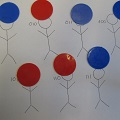Suppose you are at a party and everyone in the room is randomly given a red or black hat. On the count of 3 you can either guess your hat colour or pass. If everyone who guesses their hat colour guesses correctly, you all win 1 million dollars! We will look at the mathematics behind this problem and connections it has to binary numbers.

Outcomes:

• Determine strategies to a game, and figure out the probability of winning.
• Introduction to binary numbers.
• Change the rules of addition and get interesting results.
• Use the problem to understand computer security.

Nasty Number Tricks and Devious DivisibilityG.H. Hardy (a famous 20th Century mathematiian) wrote: 'Beauty is the first test: there is no permanent place in the world for ugly mathematics'.

Let us face it-algebra can be hard and there will be point for everyone when they find using algebra is difficult. But, believe it or not, algebra can be really beautiful and is, in fact, a powerful tool! Algebra can give a great sense of achievement every time a problem is solved and can, definitely, provide effective methods to break down and solve problems. In this talk, you will feel such a satisfaction and you will see how algebra allows you to take a situation and make it more general by examining nasty number tricks and devious divisibility.

Outcomes:

• TBA.

NimThe game of Nim is presented as an introduction to impartial game theory. Students enjoy the manipulation of tokens and often get so involved in the fun of “playing” that they do not realize that they are, in fact, doing mathematics. Binary notation is hidden behind the joy of problem solving.

Outcomes:

• Play games and analyze their strategies.
• Generalize solutions to a game.
• Recognize equivalent games.
• Try to deduce mathematical theorems.
• Introduce binary numbers.

Numeral Systems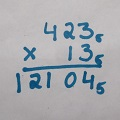Take a journey that will take you to the very first systems of numerals in ancient civilizations. Learn the needs that lead the Egyptians to develop clever arithmetic methods to fill their everyday needs, the origin of our system for measuring time and much more!

Outcomes:

• Get a better understanding of the notion of infinity.
• Look at the famous problem of Hilbert's motel.

Permutations & Combinations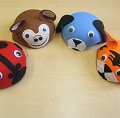How many winning poker hands are there? What is the probability in a room of 25 people that two people will share the same birthday? In this talk we will explore permutations and combinations through fun problem solving activities.

Outcomes:

• Learn to count the number of possibilities for a situation.
• Understand situations where order matters and where it doesn't.
• Use counting to understand probabilities.
• Develop fast math calculation skills.

Pi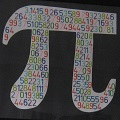We all know Pi is the ratio of a circles circumference to its diameter and that mathematicians around the world celebrate Pi day, but why else is this number so important and interesting? In this talk we will do some activities that will generate Pi in a surprising way and see why else people love Pi

Outcomes:

• Why do we care and keep looking for pi.
• How we historically estimated pi.

Prime Numbers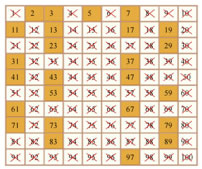Learn how ancient Greeks found prime numbers, and how huge primes today are the secret to internet encryption! We will learn some divisibility "tricks", prove that there are an infinite number of primes, and explore one of the oldest unsolved problems in mathematics: the Goldbach Conjecture!

Outcomes:

• Understand divisibility, factorization and prime numbers.
• Find solutions to problems by sieving.
• Why prime numbers are so important.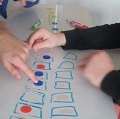Toads & Frogs is a game that is easy to play and fun to explore. We will find the number of moves needed to solve the game, and discover more about number patterns within the game.

Outcomes:

• Study the mathematics behind the strategies to a game.
• Use formulas to express number patterns.
• Introduce polynomials.

Tower of Hanoi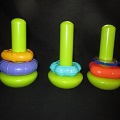The Tower of Hanoi is a very old and very popular problem. How many moves does it take to move a stack of disks from one peg to another, following certain rules? Legend has it that priests in a temple were solving the puzzle with 64 golden disks, and when the last move is completed, the world would end!

Outcomes:

• Study a classical game.
• Find number patterns within data.
• Use formulas to express number patterns.

 Many Thanks to our Partners!Copyright 2015 www.nsmathcircles.com All Rights Reserved.